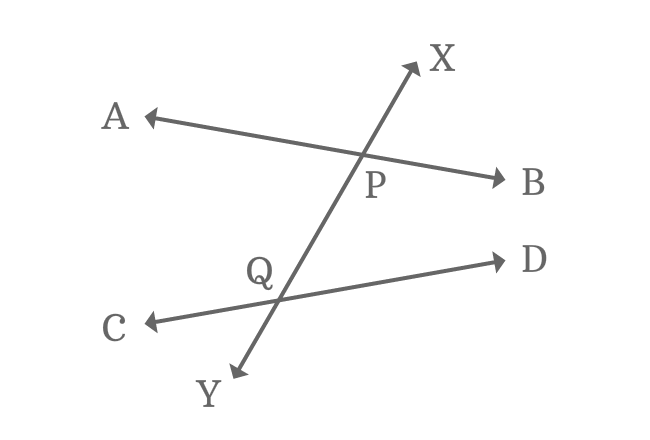# Exterior angles formed by Nonparallel lines and their TransversalExternally, some angles are formed geometrically when two or more nonparallel lines are intersected by their transversal line and the outer angles are called as exterior angles of nonparallel lines and their transversal.

Look at the picture which expresses intersection of two nonparallel lines and their transversal. In this case, four angles are formed externally and they are called as exterior angles of nonparallel lines and their transversal line.

## Exterior anglesWhen two nonparallel lines are cut by their transversal, four exterior angles are formed possibly in geometric system.

$1. \,\,\,\,\,\,$ $\angle APX$ is first external angle

$2. \,\,\,\,\,\,$ $\angle XPB$ is second external angle

$3. \,\,\,\,\,\,$ $\angle DQY$ is third external angle

$4. \,\,\,\,\,\,$ $\angle YQC$ is fourth external angle

The four angles are formed externally. So, they are known as exterior angles of nonparallel lines and their transversal.

### Property

All exterior angles are not equal when two nonparallel lines are cut by their transversal line. It is due to the non-parallelism of the intersecting lines.

A best free mathematics education website that helps students, teachers and researchers.

###### Maths Topics

Learn each topic of the mathematics easily with understandable proofs and visual animation graphics.

###### Maths Problems

A math help place with list of solved problems with answers and worksheets on every concept for your practice.

Learn solutions

###### Subscribe us

You can get the latest updates from us by following to our official page of Math Doubts in one of your favourite social media sites.